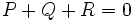# Elliptic curve group

This article defines a natural context where a group occurs, or is associated, with another algebraic, topological or analytic structure
View other occurrences of groups
This term is related to: algebraic number theory
View other terms related to algebraic number theory | View facts related to algebraic number theory

## Definition

Let$k$ be a field and$E = 0$ be the equation of an elliptic curve (viz, a nonsingular cubic curve) over$k$. Let$C$ be the locus of$E$ in the projective plane over$k$. Then, we define the elliptic curve group over$E$ in the following equivalent ways:

• It is the quotient group of the free abelian group on the set of all points of$C$, by the relations$P + Q + R = 0$ for every collinear triple of points$P,Q,R$ (here, each point is counted with multiplicity, so if a line is tangent at$P$ and also passes through$Q$, we get$2P + Q = 0$).
• It is a group whose set of points is identified with the set of points in$C$, where the identity element is the inflection (at infinity) and the sum of any three collinear points is zero.

### Equivalence of definitions

To prove the equivalence of the above definitions, we need to show that the addition defined by the collinearity relation is actually associative. This follows as a consequence of Bezout's theorem.

## Facts

### For a general cubic curve

If we remove the condition of nonsingularity, we still get a monoid analogous to the elliptic curve. The problem is at the singularity points.

### Different fields for the same curve

If$E$ is the equation of an elliptic curve with coefficients over a field$k$, then we can consider$E$ as the equation of an elliptic curve over a field$K$ containing$k$.

The elliptic curve group over this bigger field will contain the elliptic curve group over a smaller field. A category-theoretic way of putting it is that any elliptic curve with coefficients in$k$ defines a functor from fields containing$k$, to groups containing the elliptic curve group.